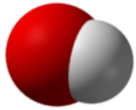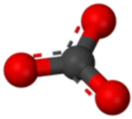# 3.8: Polyatomic Ions

$$\newcommand{\vecs}{\overset { \rightharpoonup} {\mathbf{#1}} }$$ $$\newcommand{\vecd}{\overset{-\!-\!\rightharpoonup}{\vphantom{a}\smash {#1}}}$$$$\newcommand{\id}{\mathrm{id}}$$ $$\newcommand{\Span}{\mathrm{span}}$$ $$\newcommand{\kernel}{\mathrm{null}\,}$$ $$\newcommand{\range}{\mathrm{range}\,}$$ $$\newcommand{\RealPart}{\mathrm{Re}}$$ $$\newcommand{\ImaginaryPart}{\mathrm{Im}}$$ $$\newcommand{\Argument}{\mathrm{Arg}}$$ $$\newcommand{\norm}{\| #1 \|}$$ $$\newcommand{\inner}{\langle #1, #2 \rangle}$$ $$\newcommand{\Span}{\mathrm{span}}$$ $$\newcommand{\id}{\mathrm{id}}$$ $$\newcommand{\Span}{\mathrm{span}}$$ $$\newcommand{\kernel}{\mathrm{null}\,}$$ $$\newcommand{\range}{\mathrm{range}\,}$$ $$\newcommand{\RealPart}{\mathrm{Re}}$$ $$\newcommand{\ImaginaryPart}{\mathrm{Im}}$$ $$\newcommand{\Argument}{\mathrm{Arg}}$$ $$\newcommand{\norm}{\| #1 \|}$$ $$\newcommand{\inner}{\langle #1, #2 \rangle}$$ $$\newcommand{\Span}{\mathrm{span}}$$$$\newcommand{\AA}{\unicode[.8,0]{x212B}}$$Figure $$\PageIndex{1}$$ (Credit: Huhu; Source: http://commons.wikimedia.org/wiki/File:Bella_Rosa_%2528Kordes_1981%2529.JPG(opens in new window); License: Public Domain)

### Have you ever read the story of Romeo and Juliet?

Shakespeare wrote, "A rose by any other name would smell as sweet" as part of the romantic balcony scene between Romeo and Juliet in the famous play. The two families were bitter rivals, but Juliet meant this statement to mean that she loved Romeo no matter what his name was. Some names are simple—we know Romeo mainly as Romeo. Most of us have a first name, middle name (often not used), and last name. In some cultures, names will be much more complex. The full name of the famous 20$$^\text{th}$$ century artist Pablo Picasso is Pablo Diego José Francisco de Paula Juan Neponuceno María de los Remedios Cipriano de la Santísima Trinidad Martyr Patricio Clito Ruíz y Picasso.

Many materials exist as simply binary compounds composed of a metal cation and a nonmetal anion, with each ion consisting of only one type of atom. Other combinations of atoms also exist, either as larger ionic complexes or complete molecules. Some of the most useful materials we work with contain polyatomic ions.

## Polyatomic Ions

A polyatomic ion is an ion composed of more than one atom. The ammonium ion consists of one nitrogen atom and four hydrogen atoms. Together, they comprise a single ion with a $$1+$$ charge and a formula of $$\ce{NH_4^+}$$. The carbonate ion consists of one carbon atom and three oxygen atoms, and carries an overall charge of $$2-$$. The formula of the carbonate ion is $$\ce{CO_3^{2-}}$$. The atoms of a polyatomic ion are tightly bonded together, and so the entire ion behaves as a single unit. The figures below show several models.Figure $$\PageIndex{2}$$: The ammonium ion $$\left( \ce{NH_4^+} \right)$$ is a nitrogen atom (blue) bonded to four hydrogen atoms (white). (Credit: Ben Mills (Wikimedia: Benjah-bmm27); Source: http://commons.wikimedia.org/wiki/File:Ammonium-3D-balls.png(opens in new window); License: Public Domain)Figure $$\PageIndex{3}$$: The hydroxide ion $$\left( \ce{OH^-} \right)$$ is an oxygen atom (red) bonded to a hydrogen atom. (Credit: Ben Mills (Wikimedia: Benjah-bmm27); Source: http://commons.wikimedia.org/wiki/File:Hydroxide-3D-vdW.png(opens in new window); License: Public Domain)Figure $$\PageIndex{4}$$: The carbonate ion $$\left( \ce{CO_3^{2-}} \right)$$ is a carbon atom (black) bonded to three oxygen atoms. (Credit: Ben Mills (Wikimedia: Benjah-bmm27); Source: http://commons.wikimedia.org/wiki/File:Carbonate-3D-balls.png(opens in new window); License: Public Domain)

The table below lists a number of polyatomic ions by name and by formula. The heading for each column indicates the charge on the polyatomic ions in that group. Note that the vast majority of the ions listed are anions—there are very few polyatomic cations.

$$1-$$ $$2-$$ $$3-$$ $$1+$$ $$2+$$
Table $$\PageIndex{1}$$: Common Polyatomic Ions
acetate, $$\ce{CH_3COO^-}$$ carbonate, $$\ce{CO_3^{2-}}$$ arsenate, $$\ce{AsO_3^{3-}}$$ ammonium, $$\ce{NH_4^+}$$ dimercury, $$\ce{Hg_2^{2+}}$$
bromate, $$\ce{BrO_3^-}$$ chromate, $$\ce{CrO_4^{2-}}$$ phosphite, $$\ce{PO_3^{3-}}$$
chlorate, $$\ce{ClO_3^-}$$ dichromate, $$\ce{Cr_2O_7^{2-}}$$ phosphate, $$\ce{PO_4^{3-}}$$
chlorite, $$\ce{ClO_2^-}$$ hydrogen phosphate, $$\ce{HPO_4^{2-}}$$
cyanide, $$\ce{CN^-}$$ oxalate, $$\ce{C_2O_4^{2-}}$$
dihydrogen phosphate, $$\ce{H_2PO_4^-}$$ peroxide, $$\ce{O_2^{2-}}$$
hydrogen carbonate, $$\ce{HCO_3^-}$$ silicate, $$\ce{SiO_3^{2-}}$$
hydrogen sulfate, $$\ce{HSO_4^-}$$ sulfate, $$\ce{SO_4^{2-}}$$
hydrogen sulfide, $$\ce{HS^-}$$ sulfite, $$\ce{SO_3^{2-}}$$
hydroxide, $$\ce{OH^-}$$
hypochlorite, $$\ce{ClO^-}$$
nitrate, $$\ce{NO_3^-}$$
nitrite, $$\ce{NO_2^-}$$
perchlorate, $$\ce{ClO_4^-}$$
permanganate, $$\ce{MnO_4^-}$$

The vast majority of polyatomic ions are anions, many of which end in -ate or -ite. Notice that in some cases, such as nitrate $$\left( \ce{NO_3^-} \right)$$ and nitrite $$\left( \ce{NO_2^-} \right)$$, there are multiple anions that consist of the same two elements. In these cases, the difference between the ions is in the number of oxygen atoms present, while the overall charge is the same. As a class, these are called oxoanions. When there are two oxoanions for a particular element, the one with the greater number of oxygen atoms gets the -ate suffix, while the one with the fewer number of oxygen atoms gets the -ite suffix. The four oxoanions of chlorine are as follows:

• $$\ce{ClO^-}$$, hypochlorite
• $$\ce{ClO_2^-}$$, chlorite
• $$\ce{ClO_3^-}$$, chlorate
• $$\ce{ClO_4^-}$$, perchlorate

In cases such as this, the ion with one more oxygen atom than the -ate anion is given a per- prefix. The ion with one fewer oxygen atom than the -ite anion is given a hypo- prefix.

## Summary

• Polyatomic ions contain more than one type of atom in the ion.
• The majority of polyatomic ions are anions that are named ending in -ate or -ite.
• Some anions have multiple forms and are named accordingly.

## Review

1. Write the formulas for the following ions:
1. ammonium
2. carbonate
3. sulfate
4. phosphate
2. Name the following ions:
1. $$\ce{PO_3^{3-}}$$
2. $$\ce{SiO_3^{2-}}$$
3. $$\ce{OH^-}$$
4. $$\ce{MnO_4^-}$$

3.8: Polyatomic Ions is shared under a CC BY-NC license and was authored, remixed, and/or curated by LibreTexts.